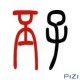# 概率与测度 (2)：积分与期望微积分现在几乎是所有专业的大一必修课了吧，在微积分课程里讲积分的时候，我们都还记得，积分就是用一堆长方形条来近似一个函数图像“下”面的面积——如右图中蓝色的那个图。这样的积分叫做黎曼积分 (Riemann Integral)，而这次要讲的积分叫做勒贝格积分 (Lebesgue Integral) ，一般最常用的示意图是右边红色的那个。从对比图中可以看到，黎曼积分是对定义域进行划分，而勒贝格积分是对值域进行划分。表面上看起来似乎是一个比较无聊的变化，但是实际上这个简单的变化使得勒贝格积分的应用范围相对于黎曼积分来说大大推广了。不过，我不知道为什么众多关于勒贝格积分的示意图都被画成我刚才展示的那张图那个样子（上面那张图来自于 wikipedia ），每次看到那张图我都会觉得非常 confusing 。左边这张图应该更为贴切一点：虽然按照值域进行了划分，但是最后得到的还是一个一个的“竖着的”（而不是像上面的图中那样“横着的”）矩形相加。比如，对于值域的某个区间 $I=[c,d]$ ，我们记（注意这只是一个记号，与 $f$ 是否有反函数没有关系）：

$f^{-1}(I) = \{x|f(x)\in I\}$

$\chi_A(x) = \begin{cases} 1 &\text{if } x \in A, \\ 0 &\text{if } x \notin A. \end{cases}$

$\phi(x) = \sum_{i=1}^n c_i\chi_{E_i}, \quad E_i=f^{-1}(\{c_i\})$

$P(\{x_1,…,x_n\}) = n$

$P(\{x_1,…,x_n\})=\sum_{i=1}^nw_ix_i$

$P(\{1\})=P(\{2\})=\ldots=P(\{6\})=\frac{1}{6}$

$\mathbb{E}\xi = \int_{[0,1]}\xi$

$\mathbb{E}\xi = \sum_{i=1}^n a_i m(E_i)$

### 18 comments to 概率与测度 (2)：积分与期望

•丕子

深入浅出 还有插图 很不错

•oldman

讲的很好，插图太赞了

•mathchain

路过赞插图:P
你画画是专门学过吗？

•pluskid

嘿嘿，不算专门学过吧，只是小时候比较喜欢画，特别是初中的时候画得比较多，其实到后来就一直没有太大的长进……

•gwang

讲得很好！

•Cola

kid有没有投今年的ICDM的论文啊？

•pluskid

没有啊

•shuufu

太给力了太给力了……

•david

请问你画图使用哪个软件？

•pluskid

请问你说的是哪个图？

•david

后面三幅，非漫画。

•pluskid

那个应该是在 wikipedia 上的图直接拿过来用的。

•xiaodada

“…,最终沦落为民科啊…”。一句话让我笑了N天，想到一次笑一次。

•Amy

你太有才了！！

•englefly

 ${x|f(x) < y_0}$可测  怎么推导出 ${x|f(x)<= y_0}$可测呢？

•pluskid

你好，取一个极限即可： $\{x|f(x)\leq y_0\}=\bigcap_{n=1}^\infty \{x|f(x) < y_0+1/n\}$

•bubin

“其实积分是被用来引入期望了，一个随机元的期望定义为

Eξ=∫[0,1]ξ”
感觉这里的积分范围改为A会好点，以免产生误解。
PS1：现在看到这个博客真是有点相见恨晚的感觉，已收藏了~
PS2：希望博主能继续写一下关于条件数学期望方面的内容，谢谢！

•张行军

作为一个非数学毕业的，在自学测度的时候，看到这篇文章，太幸运。非常谢谢。